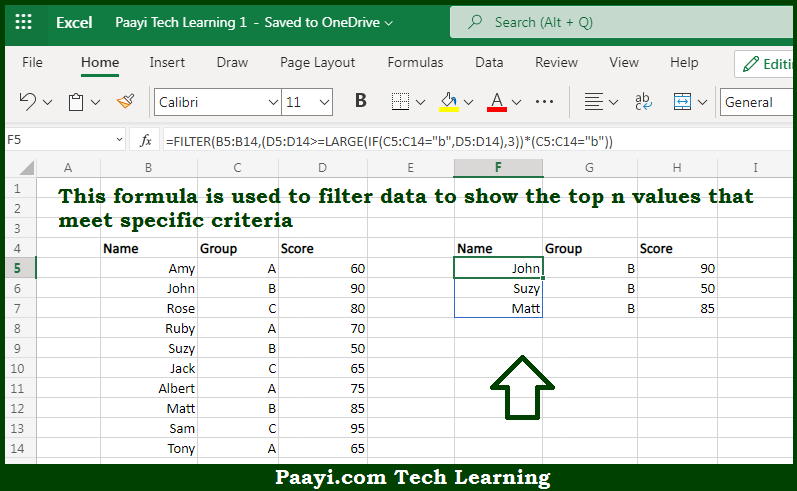# Learn How to Filter On Top n Values With Criteria in Microsoft Excel

Written by | 0 Comments | 583 Views

In this article, you will learn how to evaluate things in Dynamic Arrays with formulas in Microsoft Excel using a single/combination(s) of functions. You will also know How to Filter On Top n Values With Criteria and see the generic formula.

Learn How to Filter On Top n Values With Criteria in Microsoft Excel

The main purpose of this formula is to filter data to show the top n values that meet specific criteria. Here we will learn how to filter top n values with criteria in the given data range in the workbook in Microsoft Excel. That implies, with the help of a formula based on the FILTER, IF, and LARGE function you can able to filter data to show the top n values that meet specific criteria. So, with the help of this formula, you can able to filter top n values with criteria in the given data range in the workbook in Microsoft Excel.

General Formula to Filter On Top n Values With Criteria

=FILTER(data,(range>=LARGE(IF(criteria),n))*(criteria))

The Explanation for the Filter On Top n Values With CriteriaSo we know that with the help of the given formula above you can able to show the top n values that meet specific criteria. Here we will learn how to filter top n values with criteria in the given data range in the workbook in Microsoft Excel. As we know that the FILTER function is used to retrieve data based on a logical test utilized with the LARGE and IF functions. Moreover, the FILTER function applies criteria with the include argument. Here the criteria are constructed with Boolean logic. So, with the help of this formula, you can able to filter a set of data to show the top n values that meet specific criteria. Here we will learn how to filter top n values with criteria in the given data range in the workbook in Microsoft Excel.